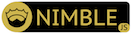units

Statically-typed quantity units.

UnitsThis module provides statically-typed units of measure. Given a unit system, any basic floating-point operations compatible with their units can be done, e.x. addition, substraction, multiplication, division, natural power. Roots can be incompatible, depending on units' powers.

Several macros with super-easy syntax are provided:

• unitSystem – creates a unit system of basic quantities
• unitQuanity – adds derivated quantities to the system
• unitAlias – provides new units, which support arbitrary functions
• unitPrefix – provides unit prefixes
• unitAbbr – provides abbreviations for units with prefixes

Both SI unit system and SI unit prefixes are available, in si and prefixes submodules respectively. Note units.si module exposes si prefixes too.

As for now, this module doesn't provide custom type mismatch communicates, but Nim's own ones suffice in most cases.

Example usage of si module and display of error-handling:

```import units.si

type Metal = object
name: string
rho: Density
cw:  Energy / (Mass * Temperature)
k:   Power / (Length * Temperature)

const copper = Metal(name: "copper",
rho:  8920.kg * 1.m^-3,
cw:    380.J / (1.kg*1.K),
k:     401.W / (1.m*1.K))

proc heating(m: Metal, E: Energy, vol: Volume): Temperature =
E / (m.cw * vol * m.rho)

echo heating(copper, 10.W * 3.s, 1.m^3)  # ok

echo heating(copper, 10.W, 1.m^3)
# FILE.nim(LINE, 13) Error: type mismatch: got (Metal, Power, Volume)
# but expected one of:
# proc heating(m: Metal; E: Energy; vol: Volume): Temperature

proc heatingErr(m: Metal, E: Energy, vol: Volume): Temperature =
E / (vol * m.rho)
# FILE.nim(LINE, 3) Error: type mismatch: got (AbsorbedDose)
# but expected 'Temperature = Si[0, 0, 0, 0, 1, 0, 0]'```

Declaring user-defined systems, quantities, units and prefixes is easy and provides (hopefully) useful error messages in case of error:

``` import units

unitSystem Imperial:  # line N
Length: yards       # line N+1
Mass:   pounds      # line N+2
Time:   seconds     # line N+3

# FILE.nim(N+1, 11) Error: no abbreviated unit name found
# for 'Length: yards', 'Length: yards(abbr)' form expected
# (maybe try 'Length: yards(y)'?)```
```import units, units.si

unitAlias:
x.yards(ya) = x * 0.9144
# FILE.nim(LINE, 19) Error: unit alias implementation
# in form expr(x).unit expected but got 'x * 0.9144'
# (maybe try '(x * 0.9144).unit'?)```

Experimental features

Experimental mode can be enabled using either via call to `unitsExperimental` template in `units` or importing `units.unitsExperimental` module.

As for now, experimental features are the following:

• value-less units operations

Example of usage:

``` import units.unitsExperimental
import units.si

let v = 10.m/s
echo v  # 10 m s^-1

# Attention! Dot binds stronger than other operators!
let area1 = 10.m^2
echo area1  # 100 m^2

let area2 = 10 * m^2
echo area2  # 10 m^2

# Units can also be binded to variables:
const m2 = m^2
let area3 = 10.m2
echo area3  # 10 m^2```

Installation

The library is available via nimble:

`nimble install units`

No initialization is needed, it's ready to use out of the box.